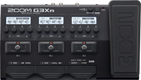# Eric Johnson - Are You Experienced?

Discussion in 'Zoom G3n/G3Xn/G5n' started by Ken Eckert, Nov 25, 2022.

1. Eric Johnson - Are You Experienced?Device: Zoom G3Xn
Firmware: 2.20

Name on device: Johnson
Optimized for: Phones/Speaker

Effects chain:This patch simulates the fat, heavy tone used on "Are You Experienced?" by Eric Johnson (not the Hendrix original). It will also work on a Zoom G1-Four with modification.

Effect: "ZNR" (Dynamics), active - "yes"
"Detect" = GTRIN
"Depth" = 100
"Threshold" = 30
"Decay" = 0

Effect: "NYC Muff" (Overdrive / Distortion), active - "yes"
"Sustain" = 80
"Tone" = 10
"Balance" = 100
"Volume" = 60

Effect: "Gt GEQ 7" (Filter), active - "yes"
"100 Hz" = 7.0
"200 Hz" = 0.0
"400 Hz" = -2.0
"800 Hz" = -4.0
"1.6 kHz" = -3.0
"3.2 kHz" = 10.0
"6.4 kHz" = 12.0
"Volume" = 90
"100 Hz" = 0.0
"200 Hz" = 0.0
"400 Hz" = 0.0
"800 Hz" = 0.0
"1.6 kHz" = 0.0
"3.2 kHz" = 0.0
"6.4 kHz" = 0.0
"Volume" = 80
"Parameter set" = A

Effect: "FD2x12" (Cabinet), active - "yes"
"MIC" = ON
"D57: D421" = 0
"Hi" = 50
"Lo" = 80

Effect: "EarlyRef" (Reverb), active - "yes"
"Decay" = 20
"Shape" = -10
"Tone" = 9
"Mix" = 30

Effect: "Church" (Reverb), active - "yes"
"PreD" = 96
"Decay" = 50
"Mix" = 25
"Tail" = On
Patch Volume: 90